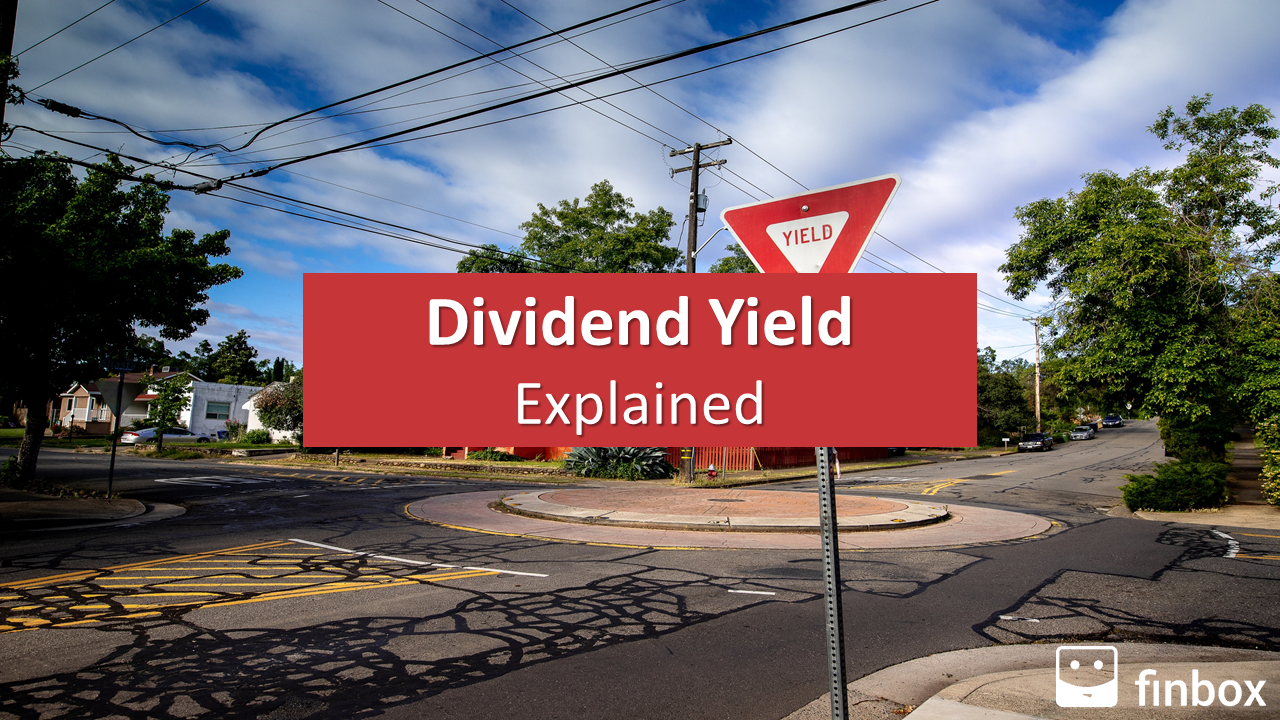### What Is The Dividend Yield?

As you learned in the first lesson of the dividend investing course, a dividend is a payment that shareholders receive for their investment in a company.

The dividend yield is a popular financial ratio that indicates the percent return you’re likely to receive in dividends on your investment. The yield is calculated by dividing the dividend per share paid by a company by the stock price per share.

If two companies have the same stock price, the company with the higher dividend will have a higher dividend yield, which means you’ll receive a higher return from dividends for each dollar you invest.

Between 1970 and 2109, 78% of the S&P 500’s cumulative return came from reinvesting dividends – that’s why the dividend yield is an essential consideration for investors.

### How To Calculate The Dividend Yield With The Right Formula?

The dividend yield is equal to the annualized dividends per share paid by a company divided by the stock price per share.

The stock price per share is the current market value of a single share of a stock.

The annual dividend per share is equal to the sum of all dividends per share paid by a company for a year.

There are several ways to calculate it but the most common method is to multiply the latest dividend by the company’s dividend frequency.

As we saw in the first lesson of the course, the dividend frequency is a financial metric that tells you how often a company pays a dividend during a year. A dividend frequency of 12 indicates that the dividend is paid 12 times a year (i.e., monthly), while a dividend frequency of four (4) indicates that the dividend is paid quarterly.

You can calculate the indicated dividend yield by multiplying the most recent dividend by the dividend frequency and dividing it by the stock price.

### Dividend Yield Example Calculation

Let’s walk through an example – we can use the aforementioned formula to calculate Apple’s (NASDAQGS:AAPL) dividend yield:

Apple’s Dividend Yield = Apple’s Annual Dividend / Apple’s Stock Price

Using the Finbox data explorer, we find the values of the formula’s components:

Based on the dividend frequency of four (4), we can conclude that Apple pays dividends quarterly. The cash amount of Apple’s last dividend was \$0.82 per share. We can calculate the annualized dividend payout by multiplying the cash amount of the last dividend by the dividend frequency.

Annualized dividend payout = Cash Amount Of Last Dividend * Dividend Frequency

\$0.82 * 4 = \$3.28

We can now calculate Apple’s dividend yield by dividing the annualized dividend payout by the stock price per share.

Apple’s Dividend Yield = \$3.28 / \$353.63 = 0.93%

Pro Tip: You don’t need to do the calculation alone and you don’t have to use a dividend yield calculator. You can use the
Finbox Data Explorer to access dividend yield data for 100,000+ companies worldwide.

### Dividend Yield: Sector Benchmark

As of July 8, 2020, the sectors with the higher dividend yields are real estate, financials, and utilities. The average dividend yield is low in many sectors since companies have cut or suspended their dividend to preserve cash and survive the coronavirus crisis.

Pro Tip: You can get up to date stats and graphs like that directly in Excel/Google Sheets using this model following the instructions inside.

### What Is A Good Dividend Yield?

A good dividend yield provides a high return on your investment but is also sustainable in the long term. Many investors think they can get huge returns by investing in high dividend yield stocks, but historically this has not been the case.

In his book, What Works On Wall Street, James O’Shaughnessy divided the entire U.S. stock market into ten (10) deciles based on dividend yields. The results of his backtest indicated that stocks in the top decile (those with the highest dividend yield) underperformed the 2nd, 3rd, and 4th deciles (those with a lower dividend yield)— the reason why – more often than not, high dividend yield stocks also come with higher risk.

Prudent investors need to consider the context around a stock’s dividend yield. Is the dividend payout sustainable based on cash flow? Does the company have a track record of increasing dividends year after year? Does the company operate in a sector with a strong headwind?

A popular list to select high dividend yield stocks is the Dividend Kings. Dividend Kings are companies that have increased their dividend for 50 or more years.

You can find the list on Finbox under Dividend Kings Portfolio in the Ideas tool and sort stocks by dividend yield, as shown below:

Read more awesome articles like this one on VintageValueInvesting.com!